×#### Thank you for registering.

One of our academic counsellors will contact you within 1 working day.

Click to Chat

1800-1023-196

+91-120-4616500

CART 0

• 0

MY CART (5)

Use Coupon: CART20 and get 20% off on all online Study Material

ITEM
DETAILS
MRP
DISCOUNT
FINAL PRICE
Total Price: Rs.

There are no items in this cart.
Continue Shopping```Algebraic Operations on Differentiation and Important Formulae

Table of Content

Algebraic Operations

Important Differential Formulae

Differentiation of trigonometric functions

Differentiation of Inverse circular function

General Theorems on Differentiation

Related Resources

Algebraic OperationsAs discussed in the previous sections, differentiation is a simple concept and we have already discussed the calculation of various functions like implicit and composite functions. In this section, we shall throw some light on algebraic operations in differentiation. Henceforth, we shall switch to the important differentiation formulae.

Yes it is possible to define algebraic operations in differentiation.

If u = f(x) and v = g(x) are differentiable functions then

(a) d/dx [f(x) + g(x)] = (d/dx) f(x) + (d/dx) g(x)

By first principle method

L.H.S. (d/dx) [f(x) + g(x)] = lim h→0 (f(x+h) + g(x+h) – g(x) – f(x))/h

= lim h→0 (f(x+h) – f(x))/h + lim h→0 (g(x+h) - g(x))/h

= (d/dx) f(x) + (d/dx) g(x)

= R.H.S.

(b) (d/dx) [f(x) – g(x)] = (d/dx) f(x) - (d/dx) g(x)

(c) Product Rule of Differentiation:

(d/dx) [f(x).g(x)] = f(x) (d/dx) g(x) + g(x) (d/dx) f(x)

In order to derive this result, proceed as follows:

Let h(x) = f(x) g(x)

⇒ h'(x) = lim h→0 (h(x + h) - h(x))/h

= lim h→0 (f(x+h) g(x+h) – f(x) g(x))/h

=  lim h→0 (f(x+h) g(x+h) – f(x+h) g(x) + f(x+h) g(x) – f(x) g(x))/h

= lim h→0 f(x+h) [g(x+h) – g(h)]/h + g(x) lim h→0 [f(x+h) – f(x)]/h

= f(x) (d/dx) g(x) + g(x) (d/dx) f(x)

= f(x) g'(x) + g(x) f'(x)

= R.H.S.

Result:

Suppose you have three functions say j(x), k(x) and l(x). Then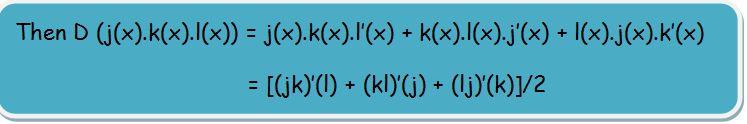Note:

This method can be generalized for product of differentiable functions as follows:

Suppose we have n functions f1, f2 , ….... , fn.

Then the derivative of the product of these n functions is given by

d/dx (f1.f2.f3. ..............  .fn) = f'1 f2 f3 ........ fn + f1 f'2 f3...... fn + f1 f2f'3 ........ fn + ....... + f1 f2 f3 ....... f'n

Illustration 1:

y = x4 + 4x3 + 5x – 6 then find dy/dx.

Solution:

dy/dx = d/dx (x4 + 4x3 + 5x – 6)

= (d/(dx) (x4) + (d/(dx) (4x3) + (d/dx) (5x) – (d/dx) (6)

= (d/dx) (x4) + 4(d/dx) (x3) + 5(d/dx) (x) - (d/(dx) (6)

= 4x3 + 12x2 + 5.1 - 0

= 4x3 + 12x2 + 5.

__________________________________________________________________________

Illustration 2:

If y = x log x – x. then find dy/dx.

Solution:

dy/dx = d/dx (x log x) - d/dx x

By product Rule on differentiation.

dy/dx = x d/dx (log x) + log x d/dx (x) - 1

= x (1)/(x) – log x .1 – 1

= – log x

(d) Quotient Rule of Differentiation:

If we have a function of the form y = (g(x)/h(x)), then the derivative is given by

D(g(x)/h(x)) = [h(x)g’(x) – g(x).h’(x)]/ h2(x)

In fact, this formula can be remembered as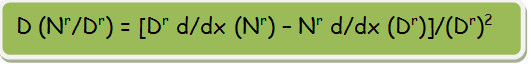Remark:

In particular, if you have a function of the form y = 1/f(x), then D(y) = – f’(x)/f2(x).

Illustration 3:

Find the differentiation coefficient of ex/log x with respect to x.

Solution:

The given function is y = ex/log x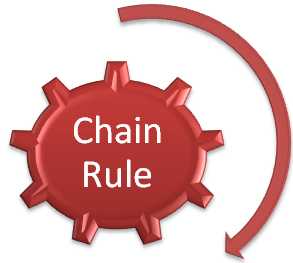dy/dx = log x . d/dx  ex - ex d/dx (log x)/(log x)2

= (log xex - ex/x)/(log x)2

= (x log xex - ex)/ x(log x)2 = (ex (x log x – 1))/(x (log x)2)

If y = f(u) and u = g(x), then dy/dx = dy/dx. du/dx = f'g(x) g'(x)

e.g. Let y = [f(x)]n. We put u = f(x). So that y = un.

Therefore, using chain rule, we get

dy/dx = dy/dx.du/dx = nu(n-1) [f'(x)](n-1) f' (x)

Having discussed various differentiation rules including product differentiation and related examples, we now switch to important formulas for differentiation:

Important Differential Formulae

First of all we begin with the differentiation of Algebraic, Exponential, Logarithmic and antilogarithmic functions and then we shall move to the trigonometric functions: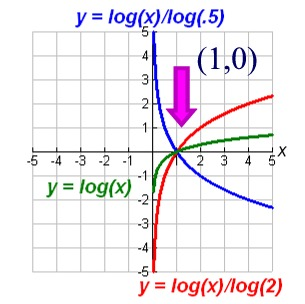1.  d/dx (xn) = nxn-1

2.  d(ex)/dx = ex

3.  d/dx (loge x) = 1/x ∀ x > 0

4. d/dx (xn) = nxn-1

5. d/dx (loga x) = 1/x logae ∀ x > 0

Differentiation of trigonometric functions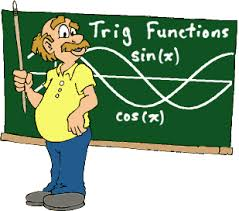1. d/dx (sin x) = cos x

2. d/dx (cos x) = - sin x

3. d/dx (tan x) = sec2x

4. d/dx (cot x) = - cosec2x

5. d/dx (sec x) = sec x tan x

6. d/dx (cosec x) = - cosec x cot x

Differentiation of Inverse circular function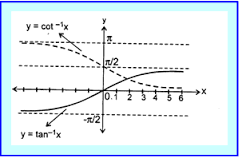1. d/dx (sin-1x) = 1/√(1 – x2); |x| < 1

2. d/dx (cos-1x) = (-1)/√(1 – x2)

3. d/dx (tan-1x) = 1/(1 + x2); x ∈ R

4. d/dx (cot-1x) = 1/(1 + x2) ; x ∈ R

5. d/dx (sec-1x) = 1/(|x|√(x2-1)); x > 1

6. d/dx (cosec-1x) = (-1) / (|x| √ (x2-1)); x > 1

General Theorems on Differentiation1. d/dx (c) = 0

2. d/dx [a f(x) + b g(x)] = af'(x) + b g'(x)

3. d/dx [f(x)g(x)] = f' (x)g(x) + f(x) g'(x)

4. d/dx [f(x)/g(x)] = (g(x) f'(x) - f(x) g'(x))/[g(x) ]2

5. d/dx [f(x)g(x) ] = f(x)g(x) [g(x)/f(x) f'(x) + g' (x) ln f(x)]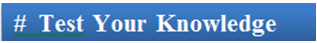Q1. (d/dx) [f(x).g(x)] =

(a) f(x) (d/dx) g(x) + g(x) (d/dx) f(x)

(b) f(x) (d/dx) g(x) + g(x) (d/dx) f(x)

(c) f(x) (d/dx) g(x) + g(x) (d/dx) f(x)

(d) f(x) (d/dx) g(x) + g(x) (d/dx) f(x)

Q2. Quotient rule of differentiation gives

(a) D[g(x)/h(x)] = [h(x)g’’(x) – g(x).h’(x)]/ h2(x)

(b) D[g(x)/h(x)] = [h’(x)g(x) – g’(x).h’(x)]/ h2(x)

(c) D[g(x)/h(x)] = [h(x)g’(x) – g(x).h’(x)]/ h(x)

(d) D[g(x)/h(x)] = [h(x)g’(x) – g(x).h’(x)]/ h2(x)

Q3. In case of differentiation of three functions f(x), g(x) and h(x),

(a) D(f(x)g(x)h(x)) = [(fg)’(h) + (gh)’(f) + (hf)’(g)]/2

(b) D(f(x)g(x)h(x)) = [(fg)’(h) + (gh)’(f) + (hf)’(g)]

(c) D(f(x)g(x)h(x)) = [(fg)(h)’ + (gh)’(f) + (hf)’(g)]/2

(d) none of the above

Q4. The derivative of the difference of two functions is

(a) The difference of product of the functions and their derivatives

(b) first function derivative of second minus second function mulitp[lied by the derivative of first.

(c) the difference of derivatives of the individual functions.

(d) can’t say

Q5. d/dx (loga x) =

(a) 1/x loge a   ∀ x > 0

(b) 1/x logae ∀ x > 0

(c) 1/x logae

(d) 1/x2 logae ∀ x > 0Q1.

Q2.

Q3.

Q4.

Q5.

(b)

(d)

(a)

(c)

(b)

Related Resources

You may wish to refer General Theorems of Differentiation.

For getting an idea of the type of questions asked, refer the previous year papers.

To read more, Buy study materials of Methods of Differentiation comprising study notes, revision notes, video lectures, previous year solved questions etc. Also browse for more study materials on Mathematics here.
```### Course Features

• 731 Video Lectures
• Revision Notes
• Previous Year Papers
• Mind Map
• Study Planner
• NCERT Solutions
• Discussion Forum
• Test paper with Video Solution